# Bivariate generalized quadratic jump diffusion processes

Building on the generalized quadratic framework (see the DiffusionRgqd package and related documentation), the template SDE for bivariate jump GQDs is given by the equation: \begin{aligned} d\mathbf{X}_t &=\boldsymbol\mu(\mathbf{X}_t,t)dt+\boldsymbol\sigma(\mathbf{X}_t,t)d\mathbf{B}_t+d\mathbf{P}_t\\ \end{aligned} where $$\mathbf{X}_t = \{X_t,Y_t\}^\prime$$, $$\mathbf{B}_t = \{B_t^{(1)},B_t^{(2)}\}^\prime$$ is a bivariate vector of independent Brownian motions, $\boldsymbol\mu(\mathbf{X}_t,t)= \begin{bmatrix} \sum_{i+j\leq 2}a_{ij}(t)X_t^iY_t^j\\ \sum_{i+j\leq 2}b_{ij}(t)X_t^iY_t^j\\ \end{bmatrix},$ $\boldsymbol\sigma(\mathbf{X}_t,t)\boldsymbol\sigma^{\prime}(\mathbf{X}_t,t) = \begin{bmatrix} \sum_{i+j\leq 2}c_{ij}(t)X_t^iY_t^j&\sum_{i+j\leq 2}d_{ij}(t)X_t^iY_t^j\\ \sum_{i+j\leq 2}e_{ij}(t)X_t^iY_t^j&\sum_{i+j\leq 2}f_{ij}(t)X_t^iY_t^j\\ \end{bmatrix},$

and $$d\mathbf{P}_t=J_{2\times q}(\mathbf{X}_t,t)d\mathbf{N}_t^{q\times 1}$$ describes a bivariate Poisson process with $$q$$-dimensional intensity vector $$\boldsymbol\lambda(\mathbf{X}_t,t)$$. Although the generalized quadratic framework allows one to calculate moment equations for jump mechanisms driven by an arbitrary number of arrival processes (i.e. $$q$$ can, in principle be any integer), DiffusionRjgqd currently provides routines for specific specifications nested within $$q=2$$. For example, for bivariate jump diffusions subject to a jump mechanism governed by a single arrival process (i.e., the jump matrix contains only a single non-zero column), we have: $\mathbf{J}(\mathbf{X}_t,t,\dot{\mathbf{z}}_t)= \begin{cases} \begin{bmatrix} \dot{z}_{11}&0\\ \dot{z}_{21}&0\\ \end{bmatrix}&\mbox{if jumps are additive}\\&\\ \begin{bmatrix} \dot{z}_{11}X_t&0\\ \dot{z}_{21}Y_t&0\\ \end{bmatrix}&\mbox{if jumps are multiplicative}\\ \end{cases},$ where the template intensity coefficient assumes the form: $\lambda^{(1)}(X_t,t) = \lambda_{00}(t)+\lambda_{10}(t)X_t+\lambda_{01}(t)Y_t,$ corresponding to the arrival rate for increments of the first element of the vector $$d\textbf{N}_t$$. Finally, it is assumed that the jump variables are distributed according to the multivariate normal distribution: $\begin{bmatrix} \dot{z}_{11}\\ \dot{z}_{12}\\ \end{bmatrix} \sim \mbox{MVN}\bigg( \begin{bmatrix} \mu_1\\ \mu_2\\ \end{bmatrix}, \begin{bmatrix} \Sigma_{11}&\Sigma_{12}\\ \Sigma_{12}&\Sigma_{22}\\ \end{bmatrix} \bigg).$ In the section that follows, we highlight some of the intricacies of analysing bivariate jump diffusions using a non-linear diffusion model.

# Multivariate jump diffusions

## A caveat on the excess factorization for multivariate processes

Although the underlying mathematics of the moment equations (see Section 1 of the vignette on transition densities) is easily extended without much complication to higher dimensions, the process of factorizing the transition density is less trivial beyond the scalar case. For example, in the bivariate case, if the jump mechanism is driven by a single arrival process ($$q=1$$), then following along the lines of the excess factorization, we can factorize the transitional density in terms of the jump free transitional density and an excess distribution just as for scalar jump diffusions. However, when the jump mechanism is driven by multiple arrival processes a number of complications arise with respect to the factorisation. For example, in the bivariate case where the jump mechanism is driven by two Poisson processes, one possible factorisation is: \begin{aligned} f_J(X_t,Y_t|X_s,Y_s) &= P(N_t^{(1)}-N_s^{(1)}=0,N_t^{(2)}-N_s^{(2)}=0) f_{D}(X_t,Y_t|X_s,Y_s)\\ &\quad+P(N_t^{(1)}-N_s^{(1)}>0,N_t^{(2)}-N_s^{(2)}>0)f_{E}(X_t,Y_t|X_s,Y_s),\\ \end{aligned} where $$f_J(X_t,Y_t|X_s,Y_s)$$ denotes the jump diffusion transition density, $$f_D(X_t,Y_t|X_s,Y_s)$$ denotes the jump free diffusion transition density and $$f_E(X_t,Y_t|X_s,Y_s)$$ again denotes the excess distribution. Although there are certainly circumstances under which the aforementioned equation would produce a valid factorisation, caution is required when applying such a factorization in the presence of multiple arrival processes: For jump diffusions with multiple jump sources, the direction of the jump variables play a critical role in characterising the dichotomy of the transitional density at short time scales. For example, for a bivariate diffusion process with a jump mechanism driven by two arrival processes (i.e., $$q=2$$), if the jump variables corresponding to the arrival process $$N_t^{(1)}$$ predict movements in a different direction to the jump variables associated with $$N_t^{(2)}$$, then the transitional density can have up to four modes over short time scales. Consequently, the excess distribution under the factorisation of the equation above will itself be multi-modal. This can be seen by considering the arrival of the first one or two jumps within a short transition horizon: Suppose the process is at $$(X_s,Y_s)$$ then arrivals due to $$N_t^{(1)}$$ and no arrivals due to $$N_t^{(2)}$$ result in a distributional mode close to the coordinate $$(X_s+\dot{z}_{11},Y_s+\dot{z}_{21})$$ (for additive jumps). On the contrary, if all arrivals are due to $$N_t^{(2)}$$ then another mode appears close to $$(X_s+\dot{z}_{21},Y_s+\dot{z}_{22})$$. For arrivals due to both $$N_t^{(1)}$$ and $$N_t^{(2)}$$ within a short time-lapse, a third mode appears close to $$(X_s+\dot{z}_{11}+\dot{z}_{21},Y_s+\dot{z}_{12}+\dot{z}_{22})$$. Were one to factorize the distribution with respect to the moments of each of these events, for example: \begin{aligned} m_{ij}^J(t) &= P(N_t^{(1)}-N_s^{(1)}=0,N_t^{(2)}-N_s^{(2)}=0) m_{ij}^D(t) \\ &\quad+P(N_t^{(1)}-N_s^{(1)}>0,N_t^{(2)}-N_s^{(2)}=0)m_{ij}^{E_1}(t) \\ &\quad+P(N_t^{(1)}-N_s^{(1)}=0,N_t^{(2)}-N_s^{(2)}>0)m_{ij}^{E_2}(t)\\ &\quad+P(N_t^{(1)}-N_s^{(1)}>0,N_t^{(2)}-N_s^{(2)}>0)m_{ij}^{E_3}(t),\\ \end{aligned} where $$E_1$$, $$E_2$$ and $$E_3$$ denotes three points of excess, one arrives at an under-determined system. This follows since the elements $$m_{ij}^{E_1}(t)$$, $$m_{ij}^{E_2}(t)$$ and $$m_{ij}^{E_3}(t)$$ cannot be determined using the jump free and jump diffusion moments alone. Fortunately, we may still use the single excess factorization when the jump mechanism implies a uni-modal excess distribution. For example, when the rows of the jump matrix share a common mean (i.e., when more than one arrival jump arrival process is present we require that, on average, jumps are made to the same location).

## A bivariate CIR process with time-inhomogeneous jump distribution

Despite the limitations of the excess factorization, one can still analyse quite interesting bivariate jump diffusions using the DiffusionRjgqd package. Consider a jump diffusion process with dynamics given by the SDE: \begin{aligned} dX_t & = (0.5(5-X_t)-0.1Y_t)dt + 0.4\sqrt{X_t}dW_t^{(1)} + dP_t^{(1)}\\ dY_t & = (0.4(6-Y_t)-0.1X_t)dt + 0.3\sqrt{Y_t}dW_t^{(2)} + dP_t^{(2)}\\ \end{aligned} subject to the initial condition $$(X_0,Y_0) = (7,7)$$, with \begin{aligned} dP_t^{(1)} &= \dot{z}_{11}dN_t^{(1)}\\ dP_t^{(2)} &= \dot{z}_{21}dN_t^{(1)}\\ \end{aligned} and $$N_t^{(1)}-N_s^{(1)}\sim\mbox{Poi}(1\times(t-s))$$. Furthermore, let: $\begin{bmatrix} \dot{z}_{12}\\ \dot{z}_{22}\\ \end{bmatrix} \sim \mbox{MVN}\bigg( \begin{bmatrix} 0.75(1+\sin(2\pi t))\\ 0.75(1+\sin(2\pi t))\\ \end{bmatrix}, \begin{bmatrix} 0.75^2(1+0.8\sin(2\pi t))^2&0\\ 0&0.75^2(1+0.8\sin(2\pi t))^2\\ \end{bmatrix} \bigg)$ where $$\mbox{MVN}(\boldsymbol\mu,\boldsymbol\Sigma)$$ denotes the multivariate Normal distribution. By defining the coefficients of the diffusion in terms of the template jump-SDE in the R environment, we can analyse the transitional density using the BiJGQD.density() function:

 library(DiffusionRjgqd)
JGQD.remove()
##  "Removed :  G0 G1 Q1 Lam0 Jmu Jsig"
 a00 = function(t){0.5*5}
a10 = function(t){-0.5}
a01 = function(t){-0.1}
c10 = function(t){0.4^2}

b00 = function(t){0.4*6}
b01 = function(t){-0.4}
b10 = function(t){-0.1}
f01 = function(t){0.3^2}

Lam00= function(t){1}

Jmu1=function(t){0.75*(1+sin(2*pi*t))}
Jmu2=function(t){0.75*(1+sin(2*pi*t))}
Jsig11=function(t){0.75^2*(1+0.8*sin(2*pi*t))^2}
Jsig22=function(t){0.75^2*(1+0.8*sin(2*pi*t))^2}

xx=seq(3,11,1/10)
yy=seq(3,11,1/10)
res= BiJGQD.density(7,7,xx,yy,0,1,1/100,Dtype='Saddlepoint')
##
##  ================================================================
##                    GENERALIZED QUADRATIC DIFFUSON
##  ================================================================
##  _____________________ Drift Coefficients _______________________
##  a00 : 0.5*5
##  a10 : -0.5
##  a01 : -0.1
##  ...   ...   ...   ...   ...   ...   ...   ...   ...   ...   ...
##  b00 : 0.4*6
##  b10 : -0.1
##  b01 : -0.4
##  ___________________ Diffusion Coefficients _____________________
##  c10 : 0.4^2
##  ...   ...   ...   ...   ...   ...   ...   ...   ...   ...   ...
##  ...   ...   ...   ...   ...   ...   ...   ...   ...   ...   ...
##  ...   ...   ...   ...   ...   ...   ...   ...   ...   ...   ...
##  f01 : 0.3^2
##  _______________________ Jump Components ________________________
##  ......................... Intensity ............................
##  Lam00 : 1
##  ........................... Jumps ..............................
##  Jmu1 : 0.75*(1+sin(2*pi*t))
##  Jmu2 : 0.75*(1+sin(2*pi*t))
##  Jsig11 : 0.75^2*(1+0.8*sin(2*pi*t))^2
##  Jsig22 : 0.75^2*(1+0.8*sin(2*pi*t))^2
## =================================================================

For purposes of the illustration we simulate trajectories for the process and superimpose the simulated paths on a contour plot of the transitional density along various points along the transition horizon. In order to illustrate the dichotomous nature of the process, we will label each simulated trajectory according to whether a jump has or has not occurred along its trajectory.

 #Simulate the process
mux     = function(x,y,t){a00(t)+a10(t)*x+a01(t)*y}
sigmax  = function(x,y,t){sqrt(c10(t)*x)}
muy     = function(x,y,t){b00(t)+b10(t)*x+b01(t)*y}
sigmay  = function(x,y,t){sqrt(f01(t)*y)}
lambda1 = function(x,y,t){Lam00(t)}
lambda2 = function(x,y,t){rep(0,length(x))}

j11     = function(x,y,z){z}
j12     = function(x,y,z){z}
j21     = function(x,y,z){z}
j22     = function(x,y,z){z}
simulate=function(x0=7,y0=7,N=10000,TT=5,delta=1/1000,pts,brks=30,plt=FALSE)
{
library(colorspace)
colpal=function(n){rev(sequential_hcl(n,power=0.8,l=c(40,100)))}

d=0                  # Time index
tt=seq(0,TT,delta)   # Time sequance
X=rep(x0,N)          # Initialize state vectors
Y=rep(y0,N)

x.traj = rep(x0,length(tt))
y.traj = rep(y0,length(tt))
x.jump = rep(0,length(tt))
y.jump = rep(0,length(tt))

# Storage for histogram snapshots:
evts = rep(0,N)
for(i in 2:length(tt))
{

X=X+mux(X,Y,d)*delta+sigmax(X,Y,d)*rnorm(N,sd=sqrt(delta))
Y=Y+muy(X,Y,d)*delta+sigmay(X,Y,d)*rnorm(N,sd=sqrt(delta))
events1 = (lambda1(X,Y,d)*delta>runif(N))
if(any(events1))
{
wh=which(events1)
evts[wh]=evts[wh]+1
X[wh]=X[wh]+j11(X[wh],Y[wh],rnorm(length(wh),Jmu1(d),sqrt(Jsig11(d))))
Y[wh]=Y[wh]+j21(X[wh],Y[wh],rnorm(length(wh),Jmu2(d),sqrt(Jsig22(d))))
}
events2 = (lambda2(X,Y,d)*delta>runif(N))

d=d+delta
if(sum(round(pts,3)==round(d,3))!=0)
{
if(plt)
{
expr1 = expression(X_t)
expr2 = expression(Y_t)
color.palette=colorRampPalette(c('green','blue','red'))
filled.contour(res$Xt,res$Yt,res$density[,,i], main=paste0('Transition Density \n (t = ',round(d,2),')'), color.palette=colpal, nlevels=41,xlab=expression(X[t]),ylab=expression(Y[t]),plot.axes= { # Add simulated trajectories points(Y~X,pch=c(20,3)[(evts>0)+1],col=c('black','red')[(evts>0)+1],cex=c(0.9,0.6)[(evts>0)+1]) if(any(events2)) { wh=which(events2) segments(xpreee[wh],ypreee[wh],X[wh],Y[wh],col='gray') } axis(1);axis(2); # Add a legend legend('topright',col=c('black','red'),pch=c(20,3), legend=c('Simulated Trajectories','Jumped')) yy=contourLines(res$Xt,res$Yt,res$density[,,i],levels=seq(0.01,0.1,length=10))
if(length(yy)>0)
{
for(j in 1:length(yy))
{
lines(yy[[j]])
}
}
})
}
}
}
}
sim=simulate(7,7,N=200,TT=0.75,delta=1/100,plt=TRUE,pts=c(0.13,0.28,0.38,0.51,0.63,0.75))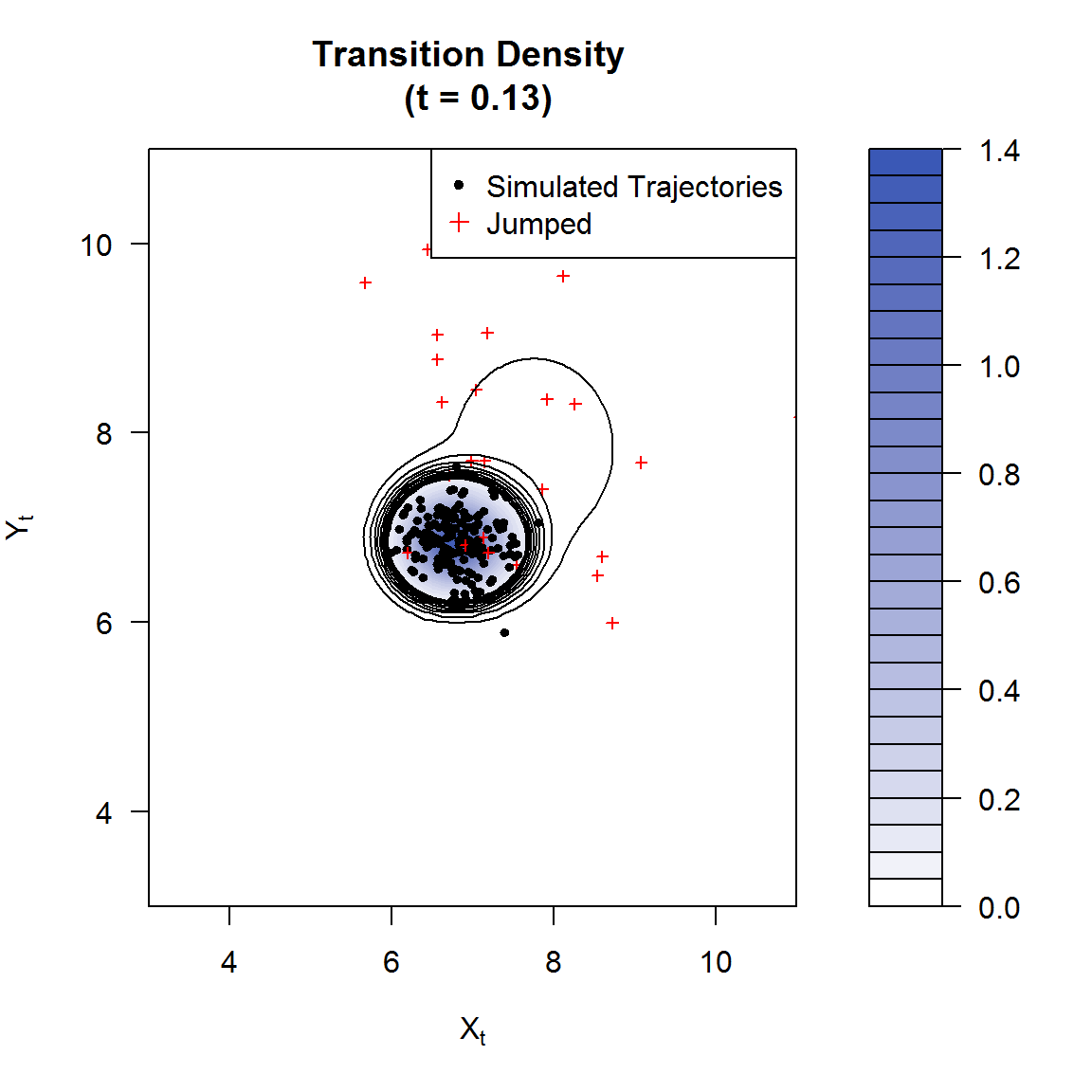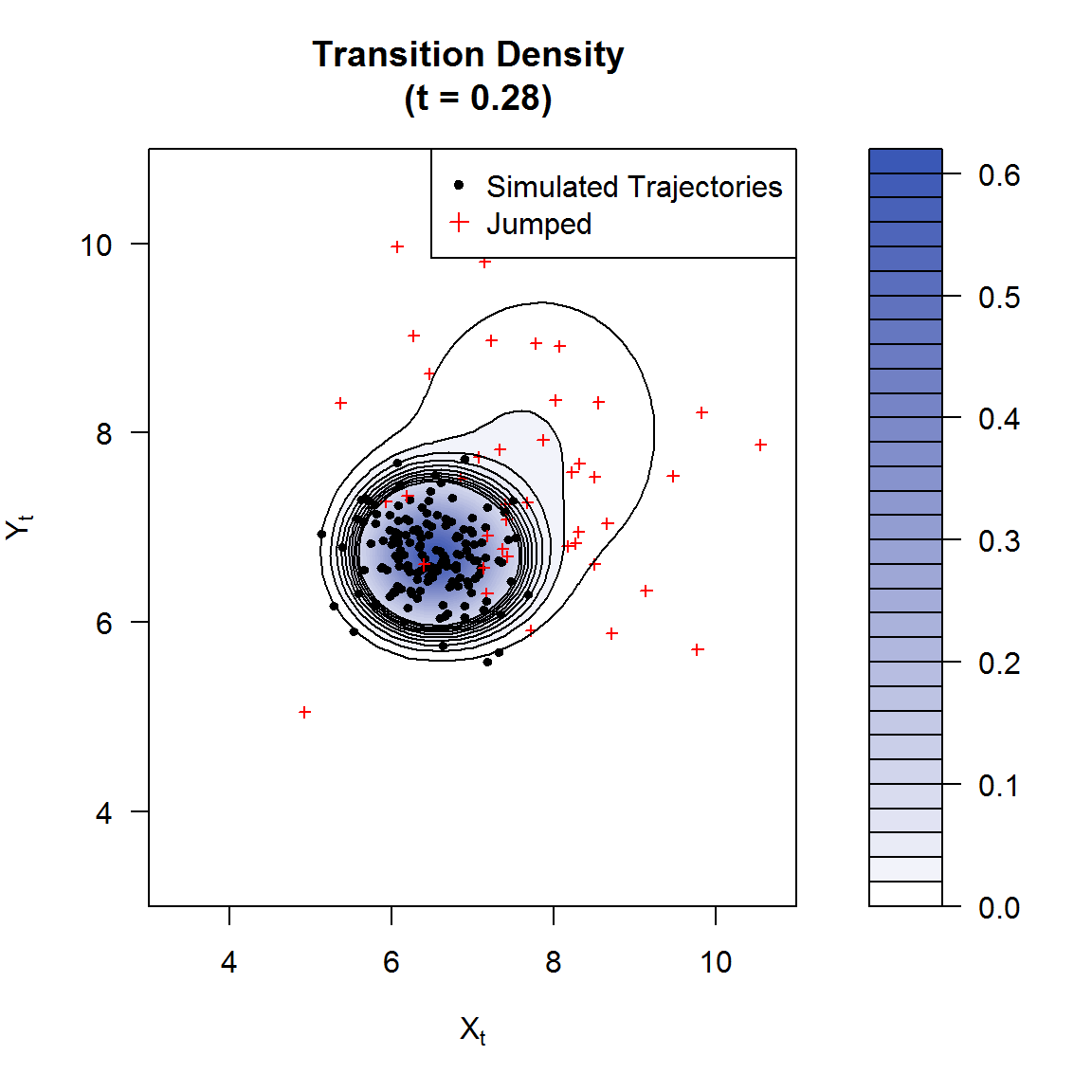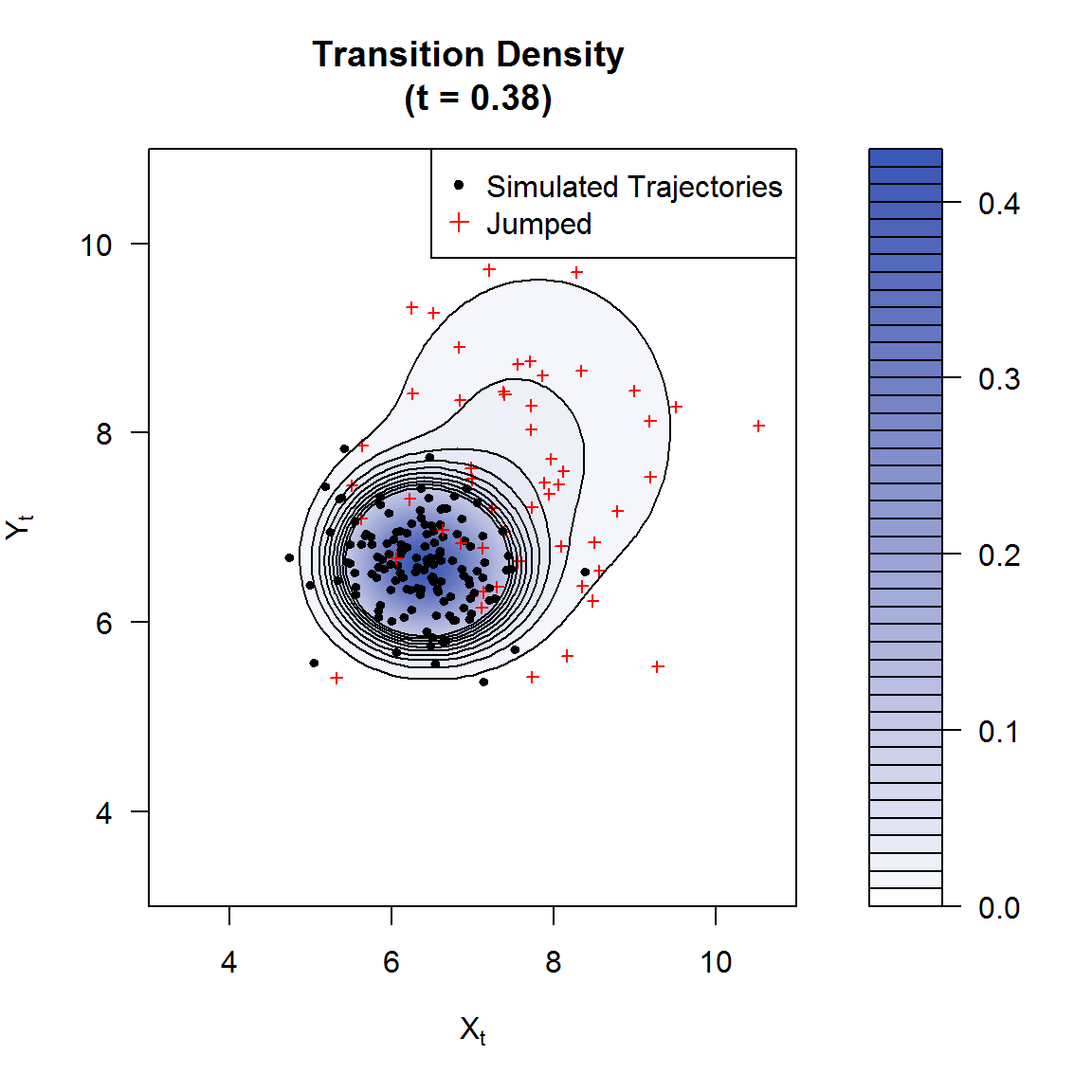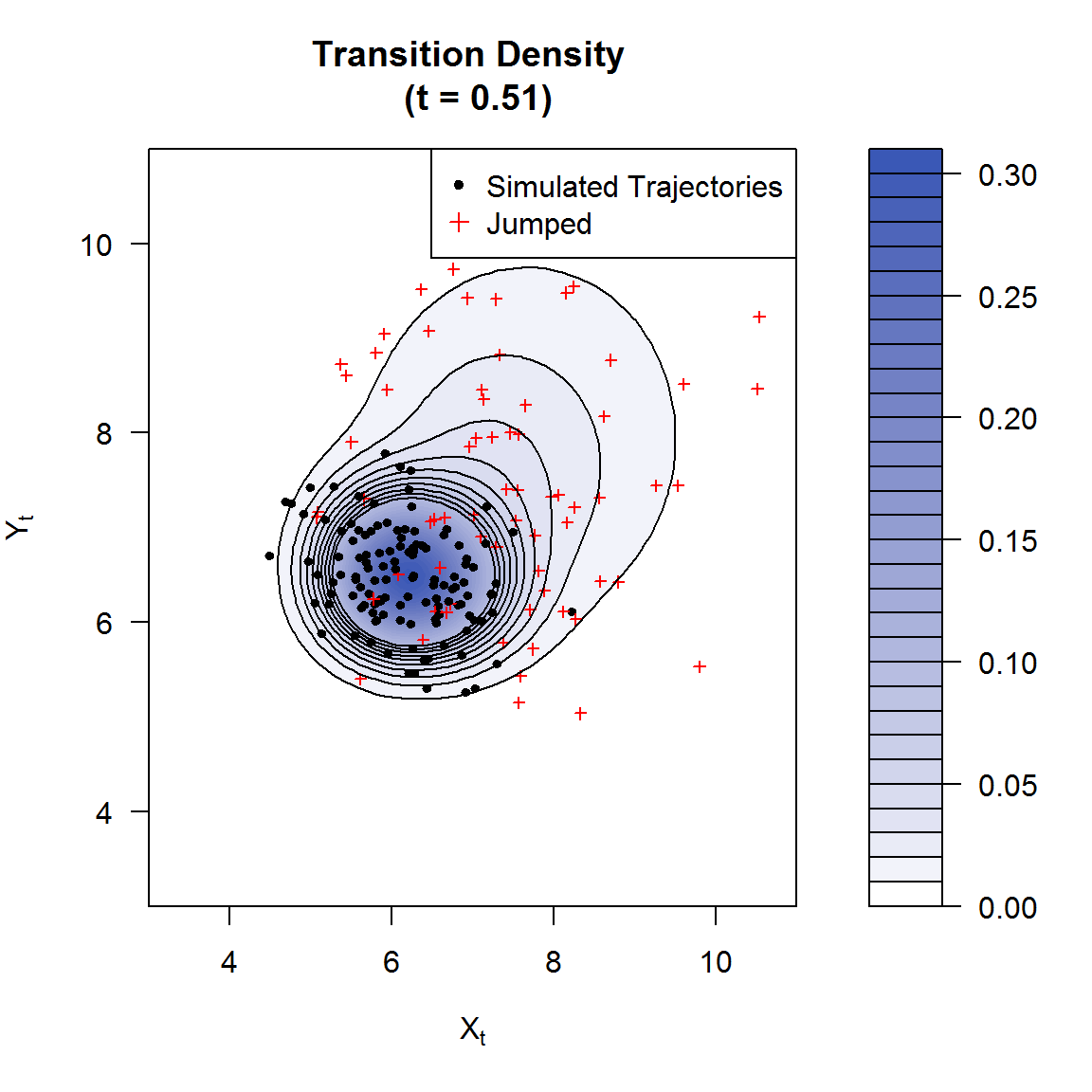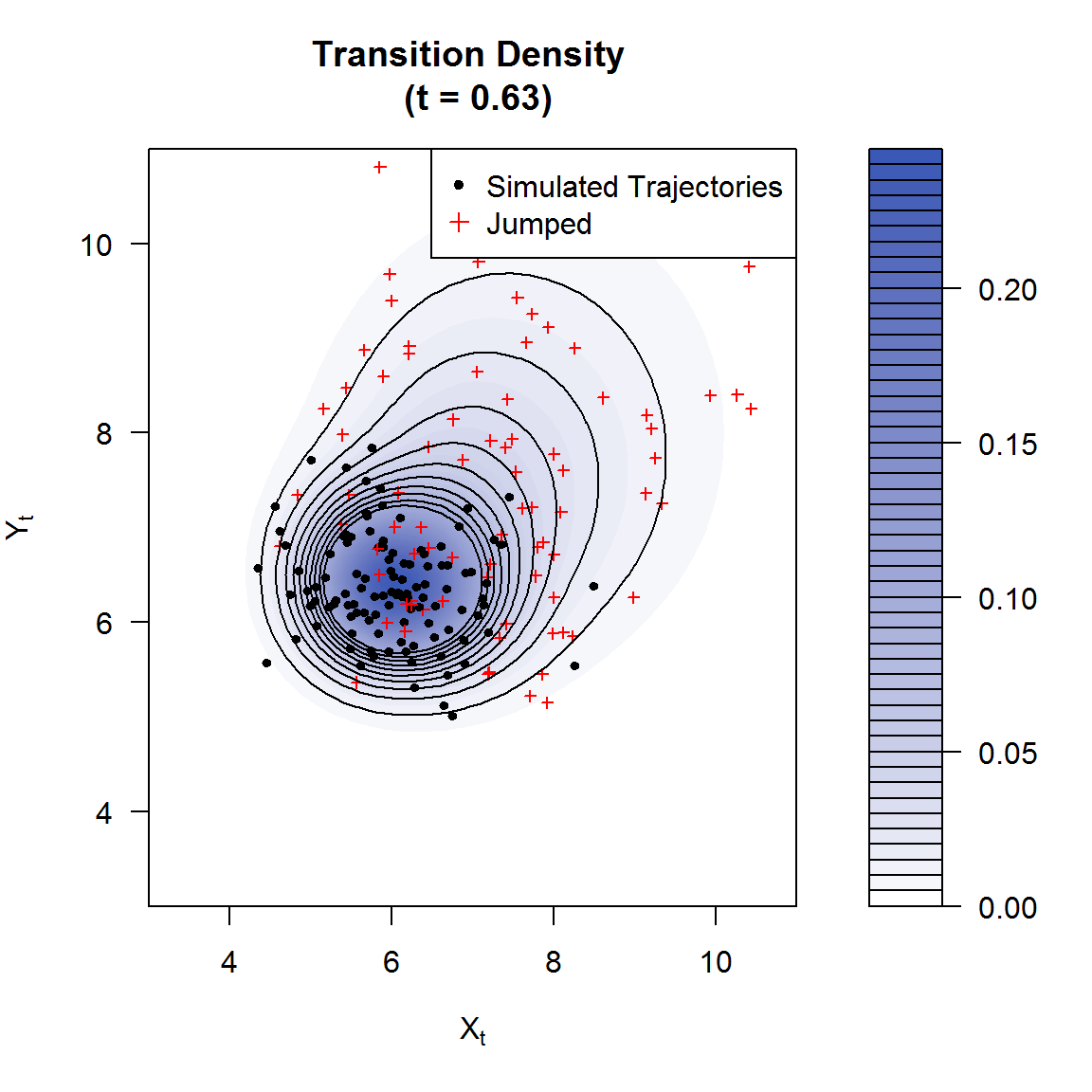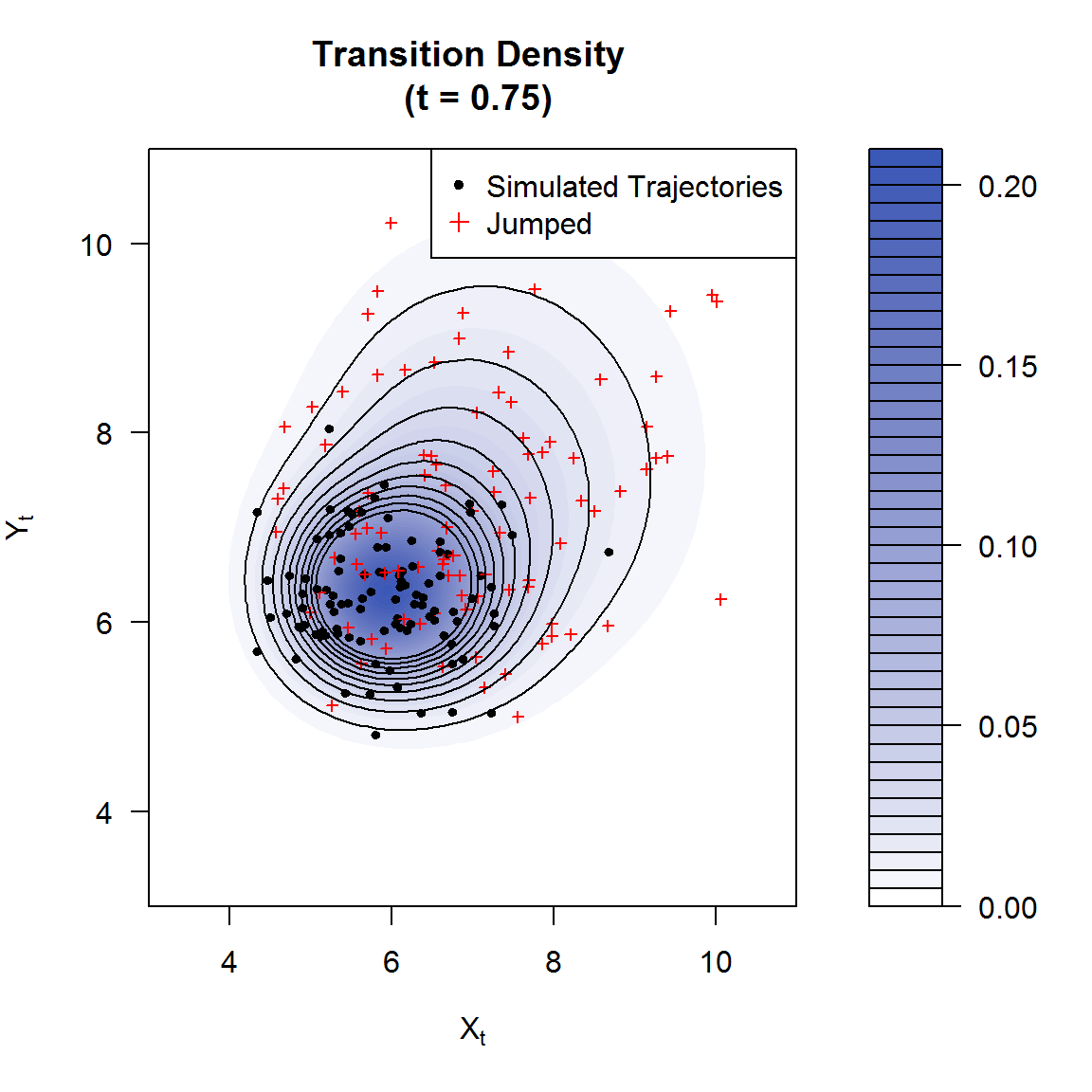During the initial stages of the evolution of the transition density, the presence of the jump variables results in a second mode some distance away from the initial values of the process. In the initial phases of the evolution of the transition density, it is clear that the dichotomy of the transition density is caused by the contrast between the diffuse and jump dynamics. Using the simulated trajectories as a guide, one can clearly see that the second mode and its surrounding area is populated predominantly by trajectories subjected to jumps, whilst the area around the primary mode of the transition density is populated mostly by purely diffuse paths. As time progresses, the contrast becomes less apparent as the jump and diffuse characteristics ‘mix’ or aggregate’, resulting in a more homogeneous distribution.

browseVignettes('DiffusionRjgqd')